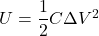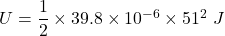## Two capacitors of 36 µF and 3.8 µF are connected parallel and charged with a 51 V power supply. Calculate the total energy stored in the two

Question

Two capacitors of 36 µF and 3.8 µF are connected parallel and charged with a 51 V power supply. Calculate the total energy stored in the two capacitors.

in progress 0
5 months 2021-09-05T11:16:41+00:00 1 Answers 0 views 0

U=0.0517 J

Explanation:

Given that

The values of capacitance

C₁ = 36 µF

C₂ =  3.8 µF

We know that when capacitor are connected in the parallel then the equivalent value of the capacitance is given as

C=C₁ +C₂

Now by putting the values

C= 36 + 3.8 µF

C = 39.8 µF

The voltage difference

ΔV= 51 V

The total stored energy in capacitor is given asNow by putting the values in the above equation we getU=0.0517 J

Therefore the stored energy in the capacitors will be 0.0517 J.